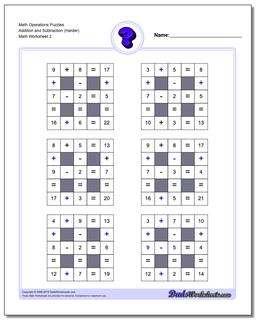# Math Worksheets: Number Grid Puzzles: Number Grid Puzzles: Math Operations Puzzles Addition and Subtraction (Harder) (Second Worksheet)## Math Operations Puzzles Addition and Subtraction (Harder) (Second Worksheet)

PropertyValue
DescriptionMath Operations Puzzles Addition and Subtraction (Harder): Math puzzle worksheets that require students to fill in operations to complete the problems with numbers near 10. (Second Worksheet)
Resource TypeWorksheet